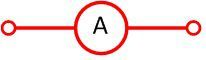# Difference Between Ammeter & Voltmeter

The major difference between the ammeter and the voltmeter is that the ammeter measures the flow of current, whereas the voltmeter measures the emf or voltage across any two points of the electrical circuit. The other differences between the ammeter and voltmeter are presented below in the comparison chart.

Electricity is measured in two ways. i.e., either through current or voltage. The current and voltage of the circuit are measured through ammeter and voltmeter. The working principle of the ammeter and voltmeter are same as that of the galvanometer.

The galvanometer uses a coil which is placed between the magnet. When the current flows through the coils, it becomes deflected. The deflection of the coils depends on the charge passing through it. This deflection is used for measuring the current or voltage. The galvanometer works as a voltmeter when the resistor is placed in series with the galvanometer.

## Comparison Chart

Basis For Comparison Ammeter Voltmeter
Definition The instruments used for measuring the current.It measures the voltage between any two points of the circuit.
Symbolic RepresentationResistance Low High
Connection It is connected in series with the circuit. It is connected in parallel with the circuit.
Accuracy More Less
Changing of Range Not possible Possible

### Definition of Ammeter

The ammeter is the measuring instruments which are used to measure the current in the circuit. It measures the small amount of current in milliamperes or micro-amperes. The ammeter is placed in series with the measuring circuit so that the whole current of the circuit passes through it.The resistance of the ammeter is very small as compared voltmeter. For ideal ammeter, the value of resistance is equal to zero. The small resistance does not obstruct the flow of current, and thus the ammeter measures the true value.

### Definition of Voltmeter

The voltmeter is the voltage measuring devices. It is connected in parallel with the electrical circuit whose potential is to be measured. The connection polarity of the voltmeter is same as that of the ammeter i.e. the positive terminal is connected to the positive polarity of the supply and the negative potential is connected to the negative polarity.

The resistance across the voltmeter is very large as compared to the ammeter. This resistance does not allow the current to flow through the voltmeter and thus the exact value of the voltage across the measuring point is measured. The value of resistance in ideal voltmeter is approximately equal to infinity.

## Key Differences between Ammeter and Voltmeter

The following are the key difference between Ammeter and Voltmeter.

1. The ammeter is defined as the device used for measuring the small value current flows in the circuit, whereas the voltmeter measures the potential difference between any two points of the electrical circuit.
2. The resistance of the ammeter is low. So that, the whole current of the circuit will pass through it. Whereas, the internal resistance of the voltmeter is very low so that the current from the circuit does not disturb the measuring of the voltmeter.
3. The ammeter is connected in series with the circuit for measuring the complete current, whereas the voltmeter is connected in parallel with the circuit. The potential difference of the parallel circuit remains same at all points. So for measuring the exact value of the potential difference, it is connected in parallel with the points whose voltage is to be measured.
4. The accuracy of the ammeter is more as compared to the voltmeter.
5. The measuring range of the voltmeter can be increases or decreases by changing the value of resistance whereas the range of ammeter can not be changed.

Nowadays, the current clamp is used for measuring the circuit current.

### 1 thought on “Difference Between Ammeter & Voltmeter”

1. Netgear Powerline support

I referred this informative article to my younger brother for his preparation for the examination and I liked the article very much. Students are benefitted through this article which promotes digital learning.